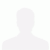Linear Buckling Analysis on Corrugated vs Normal Plate Girder

This is a Linear Buckling Analysis done on corrugated web plate girder and normal web plate girder for comparison study purpose. The model was made in Autodesk Inventor and the analysis was done in Autodesk Inventor Nastran. For this analysis, following test parameters were used: Length = 10 m hw = 1000 mm t1 = t2 = 8 mm tw = 5 mm b1 = b2 = 300 mm a1 = 100 mm a2 = 50 mm a3 = 40 mm a4 = 50 mm α = 53° Material = Mild Steel
Published 1 month ago
analysis cad simulation autodesk-inventor mechanical-engineering 3d-cad stress-analysis fusion-360 buckling-analysis linear-buckling-analysis buckling engineering-simulation inventor-nastran design-analysis